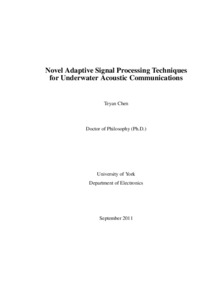# Novel Adaptive Signal Processing Techniques for Underwater Acoustic Communications

Chen, Teyan (2011) Novel Adaptive Signal Processing Techniques for Underwater Acoustic Communications. PhD thesis, University of York.Preview
Text
PhD_Thesis_by_Teyan_Chen.pdf
Available under License Creative Commons Attribution-Noncommercial-No Derivative Works 2.0 UK: England & Wales.

Download (1714Kb)

## Abstract

The underwater acoustic channel is characterized by time-varying multipath propagation with large delay spreads of up to hundreds of milliseconds, which introduces severe intersymbol interference (ISI) in digital communication system. Many of the existing channel estimation and equalization techniques used in radio frequency wireless communication systems might be practically inapplicable to underwater acoustic communication due to their high computational complexity. The recursive least squares (RLS)-dichotomous coordinate descent (DCD) algorithm has been recently proposed and shown to perform closely to the classical RLS algorithm while having a significantly lower complexity. It is therefore a highly promising channel estimation algorithm for underwater acoustic communications. However, predicting the convergence performance of the RLS-DCD algorithm is an open issue. Known approaches are found not applicable, as in the RLS-DCD algorithm, the normal equations are not exactly solved at every time instant and the sign function is involved at every update of the filter weights. In this thesis, we introduce an approach for convergence analysis of the RLS-DCD algorithm based on computations with only deterministic correlation quantities. Equalization is a well known method for combatting the ISI in communication channels. Coefficients of an adaptive equalizer can be computed without explicit channel estimation using the channel output and known pilot signal. Channel-estimate (CE) based equalizers which re-compute equalizer coefficients for every update of the channel estimate, can outperform equalizers with the direct adaptation. However, the computational complexity of CE based equalizers for channels with large delay spread, such as the underwater acoustic channel, is an open issue. In this thesis, we propose a low-complexity CE based adaptive linear equalizer, which exploits DCD iterations for computation of equalizer coefficients. The proposed technique has as low complexity as O(Nu(K+M)) operations per sample, where K and M are the equalizer and channel estimator length, respectively, and Nu is the number of iterations such that Nu << K and Nu << M. Moreover, when using the RLS-DCD algorithm for channel estimation, the computation of equalizer coefficients is multiplication-free and division-free, which makes the equalizer attractive for hardware design. Simulation results show that the proposed adaptive equalizer performs close to the minimum mean-square-error (MMSE) equalizer with perfect knowledge of the channel. Decision feedback equalizers (DFEs) can outperform LEs, provided that the effect of decision errors on performance is negligible. However, the complexity of existing CE based DFEs normally grows squarely with the feedforward filter (FFF) length K. In multipath channels with large delay spread and long precursor part, such as in underwater acoustic channels, the FFF length K needs to be large enough to equalize the precursor part, and it is usual that K > M. Reducing the complexity of CE based DFEs in such scenarios is still an open issue. In this thesis, we derive two low complexity approaches for computing CE based DFE coefficients. The proposed DFEs operate together with partial-update channel estimators, such as the RLS-DCD channel estimator, and exploit complex-valued DCD iterations to efficiently compute the DFE coefficients. In the first approach, the proposed DFE has a complexity of O(Nu l log 2l) real multiplications per sample, where l is the equalizer delay and Nu is the number of iterations such that Nu << l. In the second proposed approach, DFE has a complexity as low as O(Nu K)+O(Nu B) + O(Nu M) operations per sample, where B is the feedback filter (FBF) length and Nu << M. Moreover, when the channel estimator also exploits the DCD iterations, e.g. such as in the RLS-DCD adaptive filter, the second approach is multiplication-free and division-free, which makes the equalizer attractive for hardware implementation. Simulation results show that the proposed DFEs perform close to the RLS CE based DFE, where the CE is obtained using the classical RLS adaptive filter and the equalizer coefficients are computed according to the MMSE criterion. Localization is an important problem for many underwater communication systems, such as underwater sensor networks. Due to the characteristics of the underwater acoustic channel, localization of underwater acoustic sources is challenging and needs to be accurate and computationally efficient. The matched-phase coherent broadband matched-field (MF) processor has been previously proposed and shown to outperform other advanced broadband MF processors for underwater acoustic source localization. It has been previously proposed to search the matched phases using the simulated annealing, which is well known for its ability for solving global optimization problems while having high computational complexity. This prevents simultaneous processing of many frequencies, and thus, limits the processor performance. In this thesis, we introduce a novel iterative technique based on coordinate descent optimization, the phase descent search (PDS), for searching the matched phases. We show that the PDS algorithm obtains matched phases similar to that obtained by the simulated annealing, and has significantly lower complexity. Therefore, it enables to search phases for a large number of frequencies and significantly improves the processor performance. The proposed processor is applied to experimental data for locating a moving acoustic source and shown to provide accurate localization of the source well matched to GPS measurements.

Item Type: Thesis (PhD) The University of York > Electronics (York) uk.bl.ethos.550272 Mr Teyan Chen 22 Nov 2011 09:30 24 Jul 2018 15:19 http://etheses.whiterose.ac.uk/id/eprint/1925

You do not need to contact us to get a copy of this thesis. Please use the 'Download' link(s) above to get a copy.
You can contact us about this thesis. If you need to make a general enquiry, please see the Contact us page.